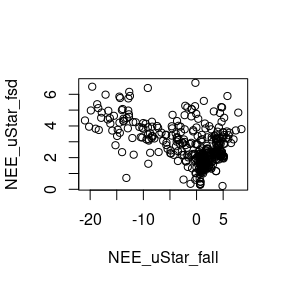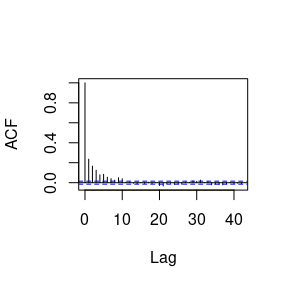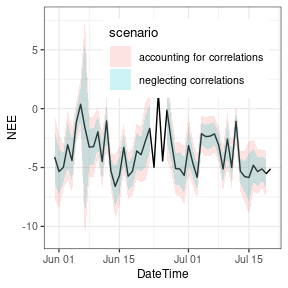# Aggregating uncertainty to daily and annual values

## Example setup

We start with half-hourly $$u_*$$-filtered and gapfilled NEE_f values. For simplicity this example uses data provided with the package and omits $$u_*$$ threshold detection but rather applies a user-specified threshold.

With option FillAll = TRUE, an uncertainty, specifically the standard deviation, of the flux is estimated for each record during gapfilling and stored in variable NEE_uStar_fsd.

library(REddyProc)
library(dplyr)
EddyDataWithPosix <- Example_DETha98 %>%
filterLongRuns("NEE") %>%
fConvertTimeToPosix('YDH',Year = 'Year',Day = 'DoY', Hour = 'Hour')
EProc <- sEddyProc$new( 'DE-Tha', EddyDataWithPosix, c('NEE','Rg','Tair','VPD', 'Ustar')) EProc$sMDSGapFillAfterUstar('NEE', uStarTh = 0.3, FillAll = TRUE)
results <- EProc$sExportResults() results_good <- results %>% filter(NEE_uStar_fqc <= 1) summary(results_good$NEE_uStar_fsd)
##     Min.  1st Qu.   Median     Mean  3rd Qu.     Max.
##  0.03535  1.69194  2.31331  2.70544  3.39922 17.19675

We can inspect, how the uncertainty scales with the flux magnitude.

results_good %>% slice(sample.int(nrow(results_good),400)) %>%
plot( NEE_uStar_fsd ~ NEE_uStar_fall, data = . )## Omitting problematic records

REddyProc flags filled data with poor gap-filling by a quality flag in NEE_<uStar>_fqc > 0 but still reports the fluxes. For aggregation we recommend computing the mean including those gap-filled records, i.e. using NEE_<uStar>_f instead of NEE_orig. However, for estimating the uncertainty of the aggregated value, the the gap-filled records should not contribute to the reduction of uncertainty due to more replicates.

Hence, first we create a column similar NEE_orig_sd to NEE_<uStar>_fsd but where the estimated uncertainty is set to missing for the gap-filled records.

results <- EProc$sExportResults() %>% mutate( NEE_orig_sd = ifelse( is.finite(.data$NEE_uStar_orig), .data$NEE_uStar_fsd, NA), NEE_uStar_fgood = ifelse( is.finite(.data$NEE_uStar_fqc <= 1), .data$NEE_uStar_f, NA) ) If the aggregated mean should be computed excluding poor quality-gap-filled data, then its best to use a column with values set to missing for poor quality, e.g. using NEE_<uStar>_fgood instead of NEE_<uStar>_f. However, the bias in aggregated results can be larger when omitting records, e.g. consistently omitting more low night-time fluxes, than with using poor estimates of those fluxes. ## Random error For a given u* threshold, the aggregation across time uses many records. The random error in each record, i.e. NEE_fsd, is only partially correlated to the random error to records close by. Hence, the relative uncertainty of the aggregated value decreases compared to the average relative uncertainty of the individual observations. ### Wrong aggregation without correlations With neglecting correlations among records, the uncertainty of the mean annual flux is computed by adding the variances. The mean is computed by $$m = \sum{x_i}/n$$. And hence its standard deviation by $$sd(m) = \sqrt{Var(m)}= \sqrt{\sum{Var(x_i)}/n^2} = \sqrt{n \bar{\sigma^2}/n^2} = \bar{\sigma^2}/\sqrt{n}$$. This results in an approximate reduction of the average standard deviation $$\bar{\sigma^2}$$ by $$\sqrt{n}$$. results %>% summarise( nRec = sum(is.finite(NEE_orig_sd)) , NEEagg = mean(NEE_uStar_f) , varSum = sum(NEE_orig_sd^2, na.rm = TRUE) , seMean = sqrt(varSum) / nRec , seMeanApprox = mean(NEE_orig_sd, na.rm = TRUE) / sqrt(nRec) ) %>% select(NEEagg, nRec, seMean, seMeanApprox) ## NEEagg nRec seMean seMeanApprox ## 1 -1.657303 10901 0.02988839 0.02650074 Due to the large number of records, the estimated uncertainty is very low. ### Considering correlations When observations are not independent of each other, the formulas now become $$Var(m) = s^2/n_{eff}$$ where $$s^2 = \frac{n_{eff}}{n(n_{eff}-1)} \sum_{i=1}^n \sigma_i^2$$, and with the number of effective observations $$n_{eff}$$ decreasing with the autocorrelation among records (Bayley 1946, Zieba 2011). The average standard deviation $$\sqrt{\bar{\sigma^2_i}}$$ now approximately decreases only by about $$\sqrt{n_{eff}}$$: $Var(m) = \frac{s^2}{n_{eff}} = \frac{\frac{n_{eff}}{n(n_{eff}-1)} \sum_{i=1}^n \sigma_i^2}{n_{eff}} = \frac{1}{n(n_{eff}-1)} \sum_{i=1}^n \sigma_i^2 \\ = \frac{1}{n(n_{eff}-1)} n \bar{\sigma^2_i} = \frac{\bar{\sigma^2_i}}{(n_{eff}-1)}$ First we need to quantify the error terms, i.e. model-data residuals. For all the records of good quality, we have an original measured value NEE_uStar_orig and modelled value from MDS gapfilling, NEE_uStar_fall. For computing autocorrelation, equidistant time steps are important. Hence, instead of filtering, the residuals of bad-quality data are set to missing. results <- EProc$sExportResults() %>%
mutate(
resid = ifelse(NEE_uStar_fqc == 0, NEE_uStar_orig - NEE_uStar_fall, NA)
,NEE_orig_sd = ifelse(
is.finite(.data$NEE_uStar_orig), .data$NEE_uStar_fsd, NA)
)

Now we can inspect the the autocorrelation of the errors.

acf(results$resid, na.action = na.pass, main = "")The empirical autocorrelation function shows strong positive autocorrelation in residuals up to a lag of 10 records. Computation of effective number of observations is provided by function computeEffectiveNumObs from package lognorm based on the empirical autocorrelation function for given model-data residuals. Note that this function needs to be applied to the series including all records, i.e. not filtering quality flag before. autoCorr <- lognorm::computeEffectiveAutoCorr(results$resid)
nEff <- lognorm::computeEffectiveNumObs(results$resid, na.rm = TRUE) c(nEff = nEff, nObs = sum(is.finite(results$resid)))
##      nEff      nObs
##  4230.522 10901.000

We see that the effective number of observations is only about a third of the number of observations.

Now we can use the formulas for the sum and the mean of correlated normally distributed variables to compute the uncertainty of the mean.

resRand <- results %>% summarise(
nRec = sum(is.finite(NEE_orig_sd))
, NEEagg = mean(NEE_uStar_f, na.rm = TRUE)
, varMean = sum(NEE_orig_sd^2, na.rm = TRUE) / nRec / (!!nEff - 1)
, seMean = sqrt(varMean)
#, seMean2 = sqrt(mean(NEE_orig_sd^2, na.rm = TRUE)) / sqrt(!!nEff - 1)
, seMeanApprox = mean(NEE_orig_sd, na.rm = TRUE) / sqrt(!!nEff - 1)
) %>% select(NEEagg, seMean, seMeanApprox)
resRand
##      NEEagg     seMean seMeanApprox
## 1 -1.657303 0.04798329   0.04254471

The aggregated value is the same, but its uncertainty increased compared to the computation neglecting correlations.

Note, how we used NEE_uStar_f for computing the mean, but NEE_orig_sd instead of NEE_uStar_fsd for computing the uncertainty.

### Daily aggregation

When aggregating daily respiration, the same principles hold.

However, when computing the number of effective observations, we recommend using the empirical autocorrelation function estimated on longer time series of residuals (autoCorr computed above) in computeEffectiveNumObs instead of estimating them from the residuals of each day.

First, create a column DoY to subset records of each day.

results <- results %>% mutate(
DateTime = EddyDataWithPosix$DateTime # take time stamp form input data , DoY = as.POSIXlt(DateTime - 15*60)$yday # midnight belongs to the previous
)

Now the aggregation can be done on data grouped by DoY. The notation !! tells summarise to use the variable autoCorr instead of a column with that name.

aggDay <- results %>%
group_by(DoY) %>%
summarise(
DateTime = first(DateTime)
,nEff = lognorm::computeEffectiveNumObs(
resid, effAcf = !!autoCorr, na.rm = TRUE)
, nRec = sum(is.finite(NEE_orig_sd))
, NEE = mean(NEE_uStar_f, na.rm = TRUE)
, sdNEE = if (nEff <= 1) NA_real_ else sqrt(
mean(NEE_orig_sd^2, na.rm = TRUE) / (nEff - 1))
, sdNEEuncorr = if (nRec <= 1) NA_real_ else sqrt(
mean(NEE_orig_sd^2, na.rm = TRUE) / (nRec - 1))
, .groups = "drop_last"
)
aggDay
## # A tibble: 365 × 7
##      DoY DateTime             nEff  nRec      NEE  sdNEE sdNEEuncorr
##    <int> <dttm>              <dbl> <int>    <dbl>  <dbl>       <dbl>
##  1     0 1998-01-01 00:30:00 11.0     21  0.124    0.760       0.536
##  2     1 1998-01-02 00:30:00  3.66     7  0.00610  1.56        1.04
##  3     2 1998-01-03 00:30:00  0        0  0.0484  NA          NA
##  4     3 1998-01-04 00:30:00  0        0  0.303   NA          NA
##  5     4 1998-01-05 00:30:00 10.9     28  0.195    0.861       0.521
##  6     5 1998-01-06 00:30:00 18.0     48  0.926    0.615       0.370
##  7     6 1998-01-07 00:30:00 18.0     48 -0.337    0.566       0.340
##  8     7 1998-01-08 00:30:00 17.7     46 -0.139    0.525       0.320
##  9     8 1998-01-09 00:30:00 17.5     45  0.614    0.474       0.290
## 10     9 1998-01-10 00:30:00 15.4     36  0.242    0.641       0.411
## # … with 355 more rowsThe confidence bounds (+-1.96 stdDev) computed with accounting for correlations in this case are about twice the ones computed with neglecting correlations.

## u* threshold uncertainty

There is also flux uncertainty due to uncertainty in u* threshold estimation. Since the same threshold is used for all times in a given uStar scenario, the relative uncertainty of this component does not decrease when aggregating across time.

The strategy is to

1. estimate distribution of u* threshold

2. compute time series of NEE (or other values of interest) for draws from this distribution, i.e. for many uStar-scenarios

3. compute each associated aggregated value

4. and then look at the distribution of the aggregated values.

Note that the entire processing down to the aggregated value has to be repeated for each uStar scenario. Hence, obtaining a good estimate of this uncertainty is computationally expensive.

1. First, we estimate many samples of the probability density of the unknown uStar threshold.

# for run-time of the vignette creation, here we use only few (3) uStar quantiles
# For real-world applications, a larger sample (> 30) is required.
nScen <- 3 # nScen <- 39

EddyDataWithPosix <- Example_DETha98 %>%
filterLongRuns("NEE") %>%
fConvertTimeToPosix('YDH',Year = 'Year',Day = 'DoY', Hour = 'Hour')
EProc <- sEddyProc$new( 'DE-Tha', EddyDataWithPosix, c('NEE','Rg','Tair','VPD', 'Ustar')) EProc$sEstimateUstarScenarios(
nSample = nScen*4, probs = seq(0.025,0.975,length.out = nScen) )
uStarSuffixes <- colnames(EProc$sGetUstarScenarios())[-1] uStarSuffixes # in real world should use > 30 ##  "uStar" "U2.5" "U50" "U97.5" 2. Produce time series of gapfilled NEE for each scenario. They are stored in columns distinguished by a suffix with the quantile. EProc$sMDSGapFillUStarScens('NEE')

3. Compute the annual mean for each scenario. Method sEddyProc_sApplyUStarScen calls a user-provided function that takes an argument suffix for each u*-threshold scenario. Here, we use it to create the corresponding NEE column name and compute mean across this column in the data exported from REddyProc.

computeMeanNEE <- function(ds, suffix){
column_name <- paste0("NEE_",suffix,"_f")
mean(ds[[column_name]])
}
FilledEddyData <- EProc$sExportResults() NEEagg <- unlist(EProc$sApplyUStarScen(computeMeanNEE, FilledEddyData))
NEEagg
##     uStar      U2.5       U50     U97.5
## -1.616926 -1.627199 -1.625671 -1.651088

4. compute uncertainty across aggregated values

sdNEEagg_ustar <- sd(NEEagg)
sdNEEagg_ustar
##  0.01462911

## Combined aggregated uncertainty

Assuming that the uncertainty due to unknown u*threshold is independent from the random uncertainty, the variances add.

sdAnnual <- data.frame(
sdRand = resRand$seMean, sdUstar = sdNEEagg_ustar, sdComb = sqrt(resRand$seMean^2 + sdNEEagg_ustar^2)
)
sdAnnual
##       sdRand    sdUstar    sdComb
## 1 0.04798329 0.01462911 0.0501638